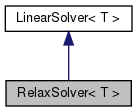Chombo + EB + MF  3.2
RelaxSolver< T > Class Template Reference

`#include <RelaxSolver.H>`

Inheritance diagram for RelaxSolver< T >:[legend]

## Public Member Functions

RelaxSolver ()

virtual ~RelaxSolver ()

virtual void setHomogeneous (bool a_homogeneous)

virtual void define (LinearOp< T > *a_op, bool a_homogeneous)

virtual void solve (T &a_phi, const T &a_rhs)Public Member Functions inherited from LinearSolver< T >
virtual ~LinearSolver ()

virtual void setConvergenceMetrics (Real a_metric, Real a_tolerance)
Set a convergence metric, along with solver tolerance, if desired. More...

## Public Attributes

bool m_homogeneous

LinearOp< T > * m_op

int m_imax

int m_normType

int m_verbosity

Real m_eps

Real m_hang

Real m_minNorm

## Detailed Description

### template<class T> class RelaxSolver< T >

Iterative solver which only uses the operator's preconditioner. Probably only useful as a bottom solver.

## ◆ RelaxSolver()

template<class T >
 RelaxSolver< T >::RelaxSolver ( )

## ◆ ~RelaxSolver()

template<class T >
 RelaxSolver< T >::~RelaxSolver ( )
virtual

## ◆ setHomogeneous()

template<class T >
 virtual void RelaxSolver< T >::setHomogeneous ( bool a_homogeneous )
inlinevirtual

Set whether the solver uses only homogeneous boundary conditions

Implements LinearSolver< T >.

## ◆ define()

template<class T >
 void RelaxSolver< T >::define ( LinearOp< T > * a_op, bool a_homogeneous )
virtual

Define the solver. a_op is the linear operator.

Implements LinearSolver< T >.

References RelaxSolver< T >::m_homogeneous, and RelaxSolver< T >::m_op.

Referenced by RelaxSolver< T >::setHomogeneous().

## ◆ solve()

template<class T >
 void RelaxSolver< T >::solve ( T & a_phi, const T & a_rhs )
virtual

Solve L(phi) = rho

Implements LinearSolver< T >.

Referenced by RelaxSolver< T >::setHomogeneous().

## ◆ m_homogeneous

template<class T >
 bool RelaxSolver< T >::m_homogeneous

public member data: whether or not to use inhomogeneous boundary conditions.

## ◆ m_op

template<class T >
 LinearOp* RelaxSolver< T >::m_op

public member data: linear operator.

Referenced by RelaxSolver< T >::define(), and RelaxSolver< T >::solve().

## ◆ m_imax

template<class T >
 int RelaxSolver< T >::m_imax

public member data: maximum number of iterations

Referenced by RelaxSolver< T >::solve().

## ◆ m_normType

template<class T >
 int RelaxSolver< T >::m_normType

public member data: which type of norm to use (default is 2-norm)

Referenced by RelaxSolver< T >::solve().

## ◆ m_verbosity

template<class T >
 int RelaxSolver< T >::m_verbosity

public member data: how much screen output user wants

Referenced by RelaxSolver< T >::solve().

## ◆ m_eps

template<class T >
 Real RelaxSolver< T >::m_eps

public member data: solver tolerance

Referenced by RelaxSolver< T >::solve().

## ◆ m_hang

template<class T >
 Real RelaxSolver< T >::m_hang

public member data: hang when min(previous residual norms) < m_hang * current residual norm (default is zero – don't check for hanging)

Referenced by RelaxSolver< T >::solve().

## ◆ m_minNorm

template<class T >
 Real RelaxSolver< T >::m_minNorm
private

Referenced by RelaxSolver< T >::solve().

The documentation for this class was generated from the following file: